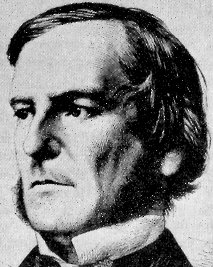Boolean functions. A function ${f:\{0,1\}^n\rightarrow\{0,1\}}$ defined on the discrete cube ${\{0,1\}^n}$ and taking its values in ${\{0,1\}}$ is called a Boolean function. Such a function encodes typically the status of a complex system with ${n}$ components. Here are two basic examples:

• ${f(x)=\mathbf{1}_{\det(x)\neq0}}$ for every ${x\in\mathcal{M}_m(\{0,1\})\equiv\{0,1\}^{m^2}}$. Here ${n=m^2}$.
• same set and ${f(x)=1}$ iff the graph with adjacency matrix ${x}$ is connected.

More generally, any property of a graph can be seen as a Boolean function by encoding the graph with its adjacency matrix, which is a Boolean object. Boolean = binary = logical = ${\{0,1\}}$. Let us recall some basic facts about Boolean calculus. For every ${b,b’\in\{0,1\}}$,

• negation operator: ${\mathbf{not}\ b = 1-b}$
• and operator: ${b\ \mathbf{and}\ b’ = bb’}$
• or operator: ${b\ \mathbf{or}\ b’ = b+b’-bb’=1-(1-b)(1-b’)}$
• equality operator: ${\mathbf{1}_{b=b’} = 1-b-b’+2bb’}$

Note: ${\mathbf{and}_{i\in I}b_i=\prod_{i\in I}b_i}$ and ${\mathbf{or}_{i\in I}b_i=1-\prod_{i\in I}(1-b_i)}$ for every ${b\in\{0,1\}^I}$.

Naive representation of Boolean functions. For every Boolean function ${f}$, if we define ${Z=\{x\in\{0,1\}^n:f(x)=0\}}$ then we have for all ${x\in\{0,1\}^n}$,

$f(x)={\mathbf{or}}_{s\not\in Z}{\mathbf{and}}_{1\leq i\leq n}(x_i=s_i) =1-\prod_{s\not\in Z}\left(1-\prod_{1\leq i\leq n}(1-s_i-x_i+2s_ix_i)\right)$

and

$f(x)={\mathbf{and}}_{s\in Z}{\mathbf{or}}_{1\leq i\leq n}(x_i\neq s_i) =\prod_{s\in Z}\left(1-\prod_{1\leq i\leq n}(1-s_i-x_i+2s_ix_i)\right).$

The left hand side formulas mean that ${f}$ can be expressed as a finite combination of the logical variables ${x_1,\ldots,x_n}$ and logical operators. In binary terms, the right hand side formulas mean that ${f}$ is a polynomial in ${x_1,\ldots,x_n}$. This is a remarkable polynomial fact! We will see that the notion of minimal cuts allows to reduce the representation complexity. On the other hand, we may observe that since ${b^2=b}$ for all ${b\in\{0,1\}}$, any polynomial in Boolean variables is identical to a polynomial of degree at most ${2}$ in each variable.

Cuts. A cut of a Boolean function ${f}$ is a couple ${(I,s)}$ where ${\varnothing\neq I\subset\{1,\ldots,n\}}$ and ${s\in\{0,1\}^I}$ such that for all ${x\in\{0,1\}^n}$ we have ${f(x)=0}$ if ${x_i=s_i}$ for all ${i\in I}$. The Boolean function identically equal to ${1}$ does not have cuts. For a Boolean function ${f}$, if ${f(x)=1}$ for some ${x\in\{0,1\}^n}$, then for any cut ${(I,s)}$ of ${f}$ we have ${x_i\neq s_i}$ for some ${i\in I}$. Conversely, if ${f(x)=0}$ for some ${x\in\{0,1\}^n}$ then there exists a cut ${(I,s)}$ of ${f}$ such that ${x_i=s_i}$ for all ${i\in I}$ (take for instance the cut ${(I,s)=(\{1,\ldots,n\},x))}$.

Representation of Boolean functions with their minimal cuts. For a Boolean function ${f}$, let us consider the partial order on its cuts defined by ${(I,s)\leq (I’,s’)}$ iff ${I\subset I’}$ and ${s_i=s’_i}$ for all ${i\in I}$. The minimal elements are called minimal cuts.

For every ${x\in\{0,1\}^n}$, we see that ${f(x)=1}$ iff for any minimal cut ${(I,s)}$ we have ${x_i\neq s_i}$ for some ${i\in I}$. Also, if ${\mathcal{S}}$ stands for the set of minimal cuts, then for all ${x\in\{0,1\}^n}$,

$f(x) =\mathbf{and}_{(I,s)\in\mathcal{S}}\mathbf{or}_{i\in I}(x_i\neq s_i) =\prod_{(I,s)\in\mathcal{S}}\left(1-\prod_{i\in I}(1-s_i-x_i+2s_ix_i)\right).$

We have taken the convention ${\prod_\varnothing=1}$. The Boolean function ${f}$ is polynomial in the Boolean variables ${x_1,\ldots,x_n}$. We already know that it can be simplified into a polynomial of degree at most two in each variable. The total degree is at most ${n}$, and this is achieved by the Boolean function ${x_1\cdots x_n}$, for which the minimal cuts are ${(\{1\},0),\ldots,(\{n\},0)}$. Note that the Boolean function ${\mathbf{1}_{x_1+\cdots+x_n\neq0}}$ has a unique minimal cut ${(\{1,\ldots,n\},(0,\ldots,0))}$, and

$\mathbf{1}_{x_1+\cdots+x_n\neq0}=1-\prod_{i=1}^n(1-x_i).$

Percolation. Let us consider the square graph obtained by taking the edges of ${\mathbb{Z}^2}$ with vertices in ${\{0,\ldots,L\}^2}$ for some fixed ${L\in\{1,2,\ldots\}}$. On each edge we put a Boolean variable and we consider the Boolean function ${f}$ equal to ${1}$ iff there exists a path with edges marked ${1}$ connecting ${(0,0)}$ with ${(L,L)}$. Could you list the minimal cuts? They give a polynomial representation of ${f}$. The number of cuts is huge, as for most complex systems.

Related. Boolean functions are called structure functions in systems reliability and engineering. Two other famous problems on Boolean functions:

Last Updated on

This site uses Akismet to reduce spam. Learn how your comment data is processed.

Syntax · Style · .